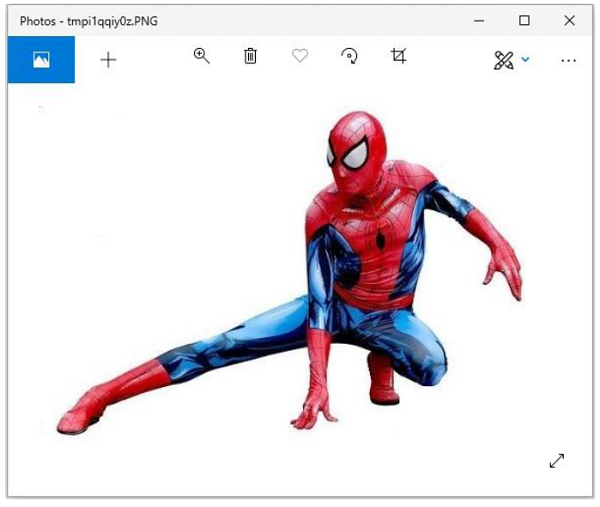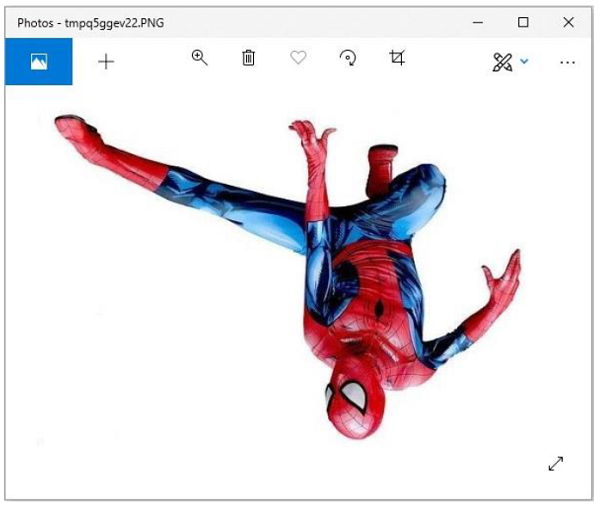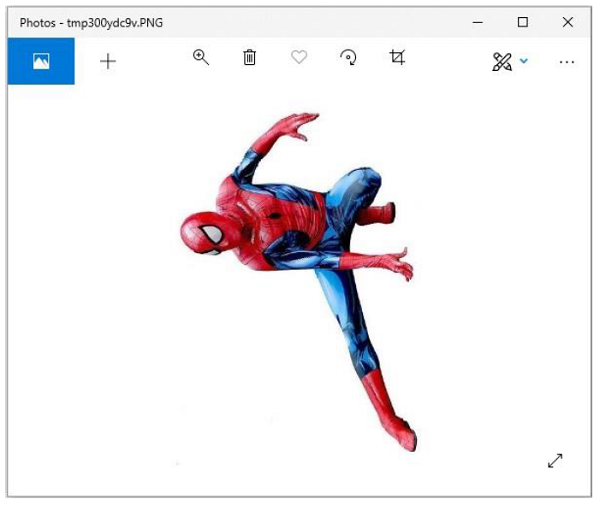# Python Pillow - Flip and Rotate Images

While working on images using python image processing library, there are instances where you need to flip an existing image to get some more insights out of it, to enhance its visibility or because of your requirement.

Image module of the pillow library allows us to flip an image very easily. We are going to use the transpose (method) function from the Image module for flipping the images. Some of the mostly commonly used methods supported by ‘transpose()’ are −

• Image.FLIP_LEFT_RIGHT − For flipping the image horizontally

• Image.FLIP_TOP_BOTTOM − For flipping the image vertically

• Image.ROTATE_90 − For rotating the image by specifying degree

### Example 1: Horizontally flipped Image

Following Python example reads an image, flips it horizontally, and displays the original and flipped image using standard PNG display utility −

```# import required image module
from PIL import Image

# Open an already existing image
imageObject = Image.open("images/spiderman.jpg")

# Do a flip of left and right
hori_flippedImage = imageObject.transpose(Image.FLIP_LEFT_RIGHT)

# Show the original image
imageObject.show()

# Show the horizontal flipped image
hori_flippedImage.show()
```

### Output

Original imageFlipped image### Example 2: Vertically Flipped Image

Following Python example reads an image, flips it vertically, and displays the original and flipped image using standard PNG display utility −

```# import required image module
from PIL import Image

# Open an already existing image
imageObject = Image.open("images/spiderman.jpg")

# Do a flip of left and right
hori_flippedImage = imageObject.transpose(Image.FLIP_LEFT_RIGHT)

# Show the original image
imageObject.show()

# Show vertically flipped image
Vert_flippedImage = imageObject.transpose(Image.FLIP_TOP_BOTTOM)
Vert_flippedImage.show()
```

### Output

Original ImageFlipped Image### Example 3: Rotate Image to a specific degree

Following Python example reads an image, rotates to a specified degree, and displays the original and rotated image using standard PNG display utility −

```# import required image module
from PIL import Image

# Open an already existing image
imageObject = Image.open("images/spiderman.jpg")

# Do a flip of left and right
hori_flippedImage = imageObject.transpose(Image.FLIP_LEFT_RIGHT)

# Show the original image
imageObject.show()

#show 90 degree flipped image
degree_flippedImage = imageObject.transpose(Image.ROTATE_90)
degree_flippedImage.show()
```

### Output

Original ImageRotated Image